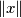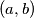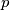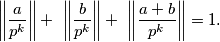IMO Shortlist 2014 problem N8

Kvaliteta:
Avg: 0,0
Težina:
Avg: 9,0

For every real number$x$, let$\lVert x \rVert$ denote the distance between$x$ and the nearest integer. Prove that for every pair$(a, b)$ of positive integers there exist an odd prime$p$ and a positive integer$k$ satisfying(Hungary)

Izvor: https://www.imo-official.org/problems/IMO2014SL.pdf